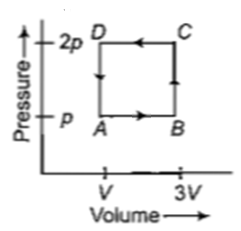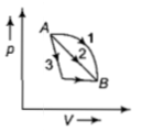You’ve reached the end of your free Videos limit.
#16 | Internal Energy (U) of Gas
(Physics) > Thermodynamics
Related Practice Questions :

A thermodynamic system is taken through the cycle ABCD as shown in figure. Heat rejected by the gas during the cycle is(a)2 pV                                     (b)4 pV

(c)$\frac{1}{2}pV$                                    (d)pV

Complete Question Bank + Test Series
Complete Question Bank

Difficulty Level:

An ideal gas goes from state A to state B

via three different processes as indicated

in the p-V diagram

If  indicates the heat absorbed

by the gas along the three processes and

indicates the change in

internal energy along the three processes

respectively, then(a)

(b)

(c)

(d)

Complete Question Bank + Test Series
Complete Question Bank

Difficulty Level:

The internal energy change in a system that has absorbed 2 kcal of heat and done 500 J of work is

(a) 8900 J                                      (b) 6400 J

(c) 5400 J                                      (d) 7900 J

Complete Question Bank + Test Series
Complete Question Bank

Difficulty Level:

A system performs work ΔW when an amount of heat is ΔQ added to the system, the corresponding change in the internal energy is ΔU. A unique function of the initial and final states (irrespective of the mode of change) is -

(1) ΔQ

(2) ΔW

(3) ΔU and ΔQ

(4) ΔU

Complete Question Bank + Test Series
Complete Question Bank

Difficulty Level:

A container of volume 1m3 is divided into two equal compartments by a partition. One of these compartments contains an ideal gas at 300 K. The other compartment is vaccum. The whole system is thermally isolated from its surroundings. The partition is removed and the gas expands to occupy the whole volume of the container. Its temperature now would be -

(1) 300 K

(2) 239 K

(3) 200 K

(4) 100 K